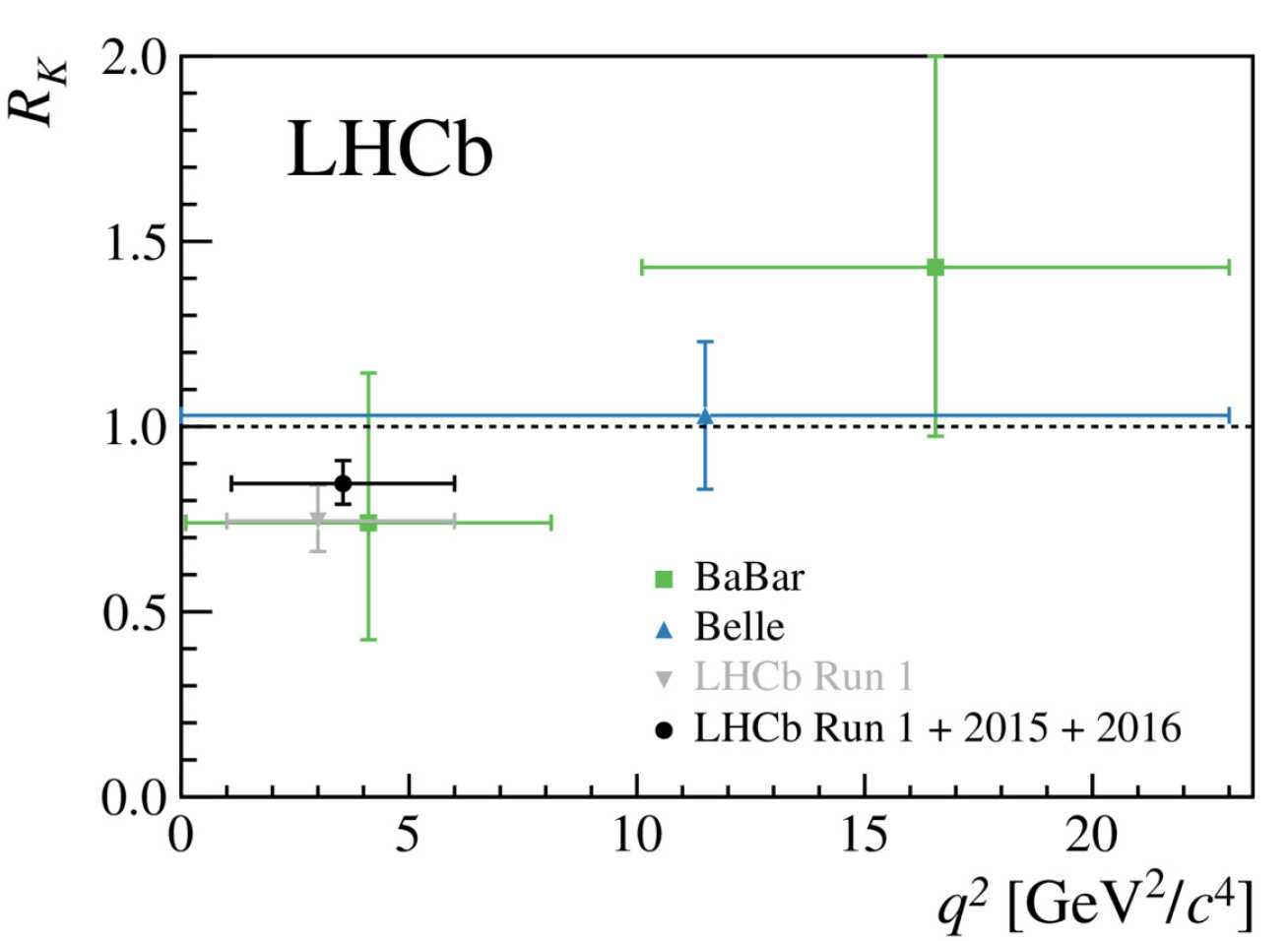# Rare Decays

Rare decays are a special class of flavour decays, where the Standard Model (SM) prediction is very small, and so New Physics effects can have very large contribution. They are decays of the form b → s(d)l⁺l⁻, a beauty quark decaying to a strange or down quark and two leptons, and are mediated by penguin and box diagrams in the SM as shown here.

It is in these decays that the strongest hints of physics beyond the standard model have been seen. Imperial are involved in the analysis of a number of rare decays. We have measured the angular distributions of the decay B⁰ → K*⁰μ⁺μ⁻, and found one of the angular distributions, P5', shows a deviation from the SM prediction, this can be seen in the figure below where the black points are the measured value, and the yellow boxes are a SM prediction. We are currently updating this measurement with the latest data.Measurement of one observable (P5') from the angular analysis of the decay B⁰ → K*⁰μ⁺μ⁻ as a function of the mass of the muon system squared, along with a prediction from the standard model in yellow.

One of the major limitations of this measurement is the theoretical uncertainties caused by interference of the more plentiful B⁰ → J/ψK*⁰ decays. To combat this we are working on an analysis that directly measures the theoretical parameters while including the effects of these charm contributions. This should probe New Physics with an especially small theoretical uncertainty.

Rare decays also allow tests of the assumption of lepton universality; that decays differing only by lepton species (electron, muon or tau) should be identical up to effects caused by their differing mass. For example the decay B⁺ → K⁺l⁺l⁻ can be studied in both the muon and electron mode at LHCb, and we have measured the value of the ratio between the two, RK. Due to their low mass electrons are complicated to reconstruct in a detector, and a lot of effort is required to understand their behaviour in LHCb. We have recently published a result that shows the ratio RK is more than two standard deviations from the expectation of lepton universality. This result can be seen in the figure below, which shows the measured value as a black point, and the prediction is the line at 1. We are now working to update this measurement with the most recent data.Measurement of the ratio of branching fractions of B⁺ → K⁺μ⁺μ⁻ and B⁺ → K⁺e⁺e⁻, predicted to be 1 in the standard model. Deviation from the prediction is seen.

In the SM, rare decays to strange quarks are more abundant (by a factor of 25) than decays to down quarks due to the structure of the CKM matrix that describes couplings between quark generations. However, there is no requirement for New Physics to obey the same structure, meaning it is possible for new particles to have an even larger relative effect in decays to down quarks. At Imperial we have measured the branching fraction of B⁺ → π⁺μ⁺μ⁻. This can be seen in the figure below, where the black points are the measurement, and the coloured boxes are different SM predictions. We are currently updating this measurement with the latest data. We can also use this measurement with the measurement of the decay to strange quarks to probe the values of the CKM matrix in loop decays.The branching fraction of the decay B⁺ → π⁺μ⁺μ⁻ as a function of the mass of the muon system squared, along with the standard model predictions from various theory groups.